In this post I will review a series of papers by Argyres, Lotito, Lü and Martone published in recent years. The main problem is to classify some four-dimensional $\mathcal{N}=2$ SCFTs, based on the possible geometries that can appear on the moduli space of vacua. Finding a geometry does not mean that a corresponding theory exists, but the complete classification provides constraints on the possible theories that can exist.

## The classification

The authors focus here on the Coulomb branch (CB), and for simplicity they restrict their attention to the rank 1 case, by which they mean that the CB has complex dimension 1. The classification proceeds in two steps.

1. First, determine the geometries that can be a CB. As shown by Seiberg and Witten a long time ago, the CB can contain singularities, where the theory has additional massless states. If the theory is conformal, the geometry must be scale invariant, and this implies that
• There can be at most one singular point.
• Any component of the CB is a flat cone, with deficit angle $\delta$.
• The singular point, which is the tip of the cone, represents the conformal vacuum (while other points are vacua where conformal invariance is spontaneously broken).
• Supersymmetry implies that the CB is special Kähler.
• The CB is supposed to be freely generated, so the CB geometry is "planar" (the complex structure is that of $\mathbb{C}$).
Using these assumptions, the conical geometries have been classified by Kodaira, and there are seven possibilities, called $II^*$, $III^*$, $IV^*$, $I_0^*$, $IV$, $III$ and $II$, each corresponding to a finite deficit angle $0 < \delta < 2\pi$. In addition, one should mention the singularities $I^*_n$ and $I_n$ with deficit angle $2 \pi$ chich correspond to cusps and are not scale invariant. In the Table below, taken from , we see indeed a scale $\Lambda$ in the equation of the curve in those cases.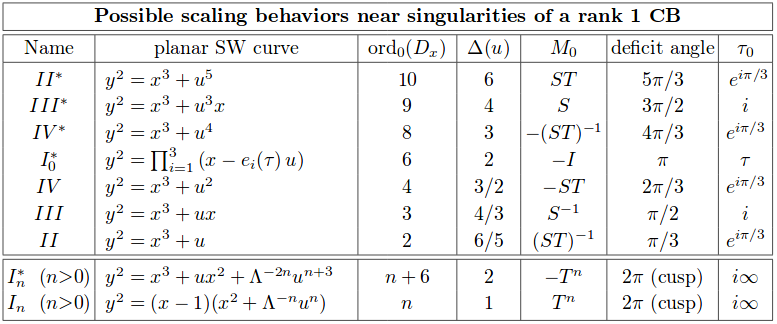2. The second step is to identify the possible $\mathcal{N}=2$ deformations of the CB geometry, which split the singularity. The physical reason for that is that several SCFTs can have the same CB geometry, but different (mass) deformations (for instance, $\mathfrak{su}(2)$ gauge theory with 4 flavors and $\mathfrak{su}(2)$ gauge theory with adjoint matter have the same CB geometry, but distinct mass deformations).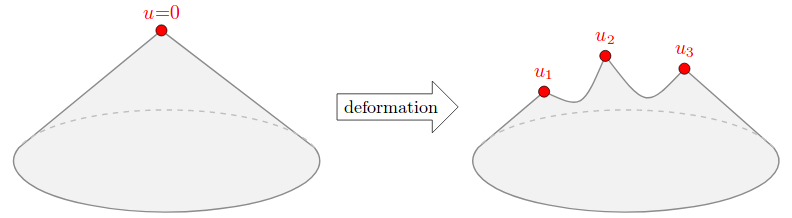The crucial point here is to take into account the restrictions imposed by supersymmetry on the possible deformations. The authors make and motivate several assumptions that I don't discuss here, and obtain a final list of 28 geometries, as reproduced here from :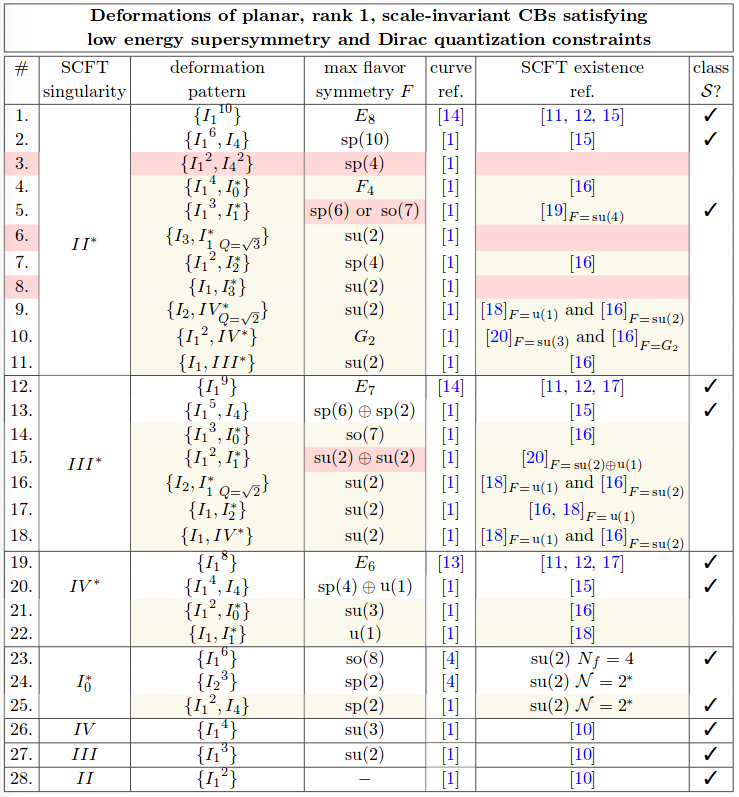A priori, the classification strategy given above can fail at different points:

• A given CB geometry can correspond to no SCFT ("non-existence")
• A given CB geometry can correspond to several SCFTs, which for instance differ only in their spectrum of irrelevant operators ("non-unicity").

## Non freely-generated CBs

The classification of  given in the previous paragraph makes the crucial assumption that the CB is holomorphically isomorphic to $\mathbb{C}$, or equivalently that the CB is freely-generated. In , the authors examine what happens if this assumption is dropped, finding new internally consistent theories.

The most general CB geometry for an $\mathcal{N}=2$ SCFT is a bouquet of $N$ cones sharing a common tip. We have $$N>1 \Longrightarrow \mbox{The CB is not freely-generated} \, .$$ Under deformation, the CB becomes a non-compact genus $g$ Riemann surface with metric singularities (indicated as before by red dots, image taken from ):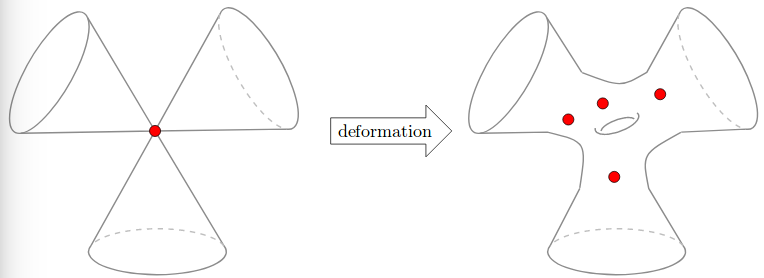Unitarity requires the scaling dimension of scalar operators to be $\geq 1$, and when the CB coordinate $u$ is the vev of such an operator, this translates into $\Delta (u) \geq 1$. But in presence of relations among the CB chiral ring generators, the coordinate is generically not the vev of an operator in the spectrum, and the bound no longer holds. When the constraint $\Delta (u) \geq 1$ is dropped, there are many more scale invariant conical geometries (Table taken from ):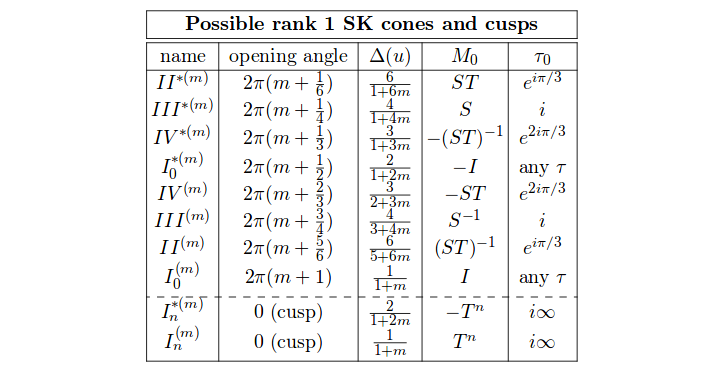Now we have infinite families parametrized by $m \in \mathbb{N}$, and when $m>0$ the singularities are said to be of irregular type.

The main result of  is that SCFTs with non-freely-generated rank 1 CBs flow to exotic fixed point theories with non-freely-generated CB chiral rings, and therefore form a closed subset of $\mathcal{N}=2$ theories under RG flow. This might explain why these have not been encountered until recently.

## Disconnected Gauge Groups

Finally, in  the authors look at what happens when gauging a discrete subgroup of symmetry in 4d $\mathcal{N}=2$ theories from the classification above. In that work, a CB geometry is denoted $[K,F]$ where $K$ is the Kodaira type, and $F$ is the flavor symmetry given in the Table above. Also, one indicates the presence of a chiral deformation parameter of scaling dimension $\delta$ by $\chi_{\delta}$. Then, one can gauge discrete automorphisms of the symmetry $F$. For instance, the theory $[I_0^*,D_4 \chi_0]$ (which is nothing but the $\mathfrak{su}(2)$ theory with 4 flavors) gives birth, gauging a $\mathbb{Z}_2$, to a theory $[III^*,B_3]$, and gauging a $\mathbb{Z}_3$ to $[II^*,G_2]$. Then one can study the RG flows between these theories, and obtain the following Table, taken from :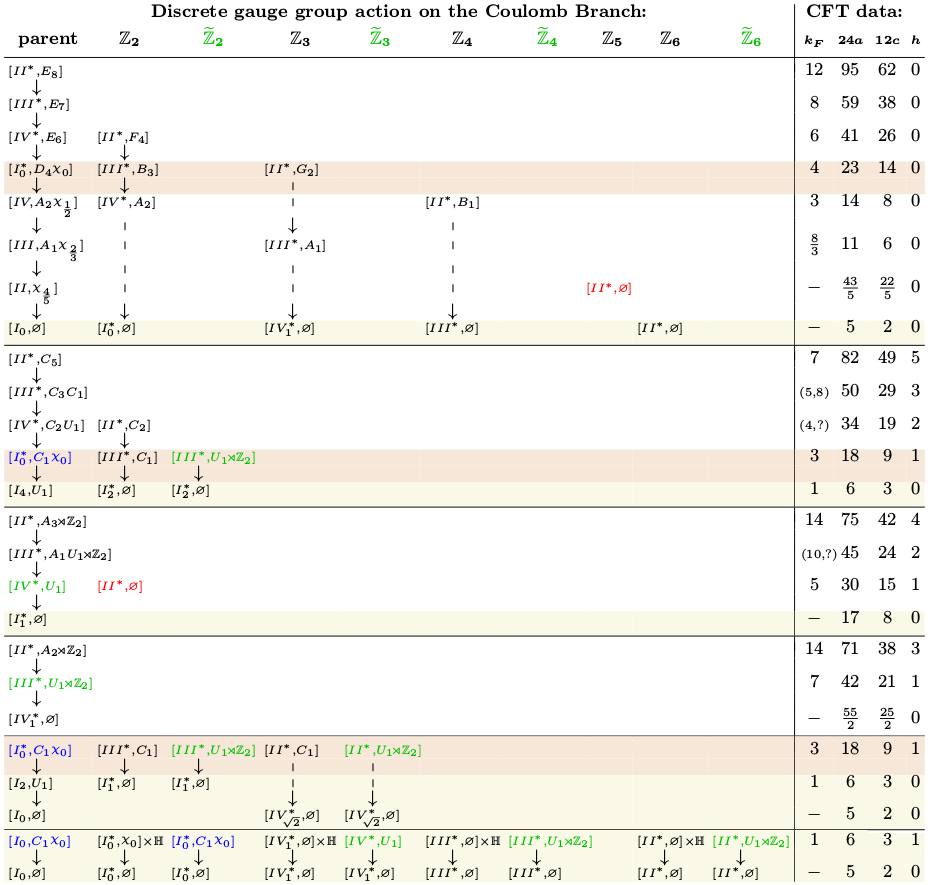In many cases, the gauging of the discrete symmetry must be combined with an appropriate gauging of R-symmetry and $SL(2,\mathbb{Z})$ transformation in order to preserve $\mathcal{N}=2$ supersymmetry. Note that a given geometry can appear several times in the Table, this just means, as noted previously, that a given CB geometry can correspond to different theories (and in this case, it can correspond to a parent theory and a daughter theory).

Importantly, as far as I understand, all this discrete gaugings are still holomorphically equivalent to $\mathbb{C}$, and therefore freely-generated. In that sense, it is something different than what is done in .

## References

 Philip Argyres, Matteo Lotito, Yongchao Lü, Mario Martone, Geometric constraints on the space of N=2 SCFTs I: physical constraints on relevant deformations, arXiv:1505.04814

 Philip C. Argyres, Yongchao Lü, Mario Martone, Seiberg-Witten geometries for Coulomb branch chiral rings which are not freely generated, arXiv:1704.05110

 Philip C. Argyres, Mario Martone, 4d $\mathcal{N}=2$ theories with disconnected gauge groups, arXiv:1611.08602

 Antoine Bourget, Alessandro Pini, Diego Rodriguez-Gomez, The Importance of Being Disconnected, A Principal Extension for Serious Groups, arXiv:1804.01108